#### 相似文章

24 四月 2018, 14:42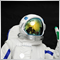0
2 639

### 开发图形界面

```//+------------------------------------------------------------------+
//|                                                      Program.mqh |
//|                                  版权所有 2018, MetaQuotes 软件公司 |
//|                                              http://www.mql5.com |
//+------------------------------------------------------------------+
//--- 用于创建图形界面的库类
#include <EasyAndFastGUI\WndEvents.mqh>
//+------------------------------------------------------------------+
//| 用于开发应用程序的类                                                 |
//+------------------------------------------------------------------+
class CProgram : public CWndEvents
{
};
```

EasyAndFast 函数库显示为单一块 (GUI 函数库), 以免令图像混乱。 您可以在 函数库页面 上查看它的完整内容。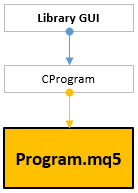CProgram 类中应该创建类似的方法来连接 MQL 程序的主要函数。 我们需要来自 OnTesterXXX() 类别中的方法来处理帧。

```class CProgram : public CWndEvents
{
public:
//--- 初始化/逆初始化
bool              OnInitEvent(void);
void              OnDeinitEvent(const int reason);
//--- "新逐笔报价" 事件处理器
void              OnTickEvent(void);
//--- 交易事件处理器
//--- 计时器
void              OnTimerEvent(void);
//--- 测试器
double            OnTesterEvent(void);
void              OnTesterPassEvent(void);
void              OnTesterInitEvent(void);
void              OnTesterDeinitEvent(void);
};
```

```//--- 包含应用程序类
#include "Program.mqh"
CProgram program;
//+------------------------------------------------------------------+
//| 智能系统初始化函数                                                   |
//+------------------------------------------------------------------+
int OnInit(void)
{
//--- 初始化程序
if(!program.OnInitEvent())
{
::Print(__FUNCTION__," > 初始化失败!");
return(INIT_FAILED);
}
//---
return(INIT_SUCCEEDED);
}
//+------------------------------------------------------------------+
//| 智能系统逆初始化函数                                                 |
//+------------------------------------------------------------------+
void OnDeinit(const int reason) { program.OnDeinitEvent(reason); }
//+------------------------------------------------------------------+
//| 智能系统逐笔报价函数                                                 |
//+------------------------------------------------------------------+
void OnTick(void) { program.OnTickEvent(); }
//+------------------------------------------------------------------+
//| 计数器函数                                                         |
//+------------------------------------------------------------------+
void OnTimer(void) { program.OnTimerEvent(); }
//+------------------------------------------------------------------+
//| ChartEvent 函数                                                   |
//+------------------------------------------------------------------+
void OnChartEvent(const int id,const long &lparam,const double &dparam,const string &sparam)
{ program.ChartEvent(id,lparam,dparam,sparam); }
//+------------------------------------------------------------------+
//| Tester 函数                                                       |
//+------------------------------------------------------------------+
double OnTester(void) { return(program.OnTesterEvent()); }
//+------------------------------------------------------------------+
//| TesterInit 函数                                                   |
//+------------------------------------------------------------------+
void OnTesterInit(void) { program.OnTesterInitEvent(); }
//+------------------------------------------------------------------+
//| TesterPass 函数                                                   |
//+------------------------------------------------------------------+
void OnTesterPass(void) { program.OnTesterPassEvent(); }
//+------------------------------------------------------------------+
//| TesterDeinit 函数                                                 |
//+------------------------------------------------------------------+
void OnTesterDeinit(void) { program.OnTesterDeinitEvent(); }
//+------------------------------------------------------------------+
```

• 控件窗体。
• 指定将要在图形上显示的余额数额的字段。
• 调整优化结果重复显示速度的字段。
• 启动重复显示的按钮。
• 结果统计表。
• 显示 EA 外部参数的表格。
• 余额曲线图形。
• 优化结果图形。
• 显示附加摘要信息的状态栏。
• 进度条显示重新滚动时总显示结果的百分比。

```class CProgram : public CWndEvents
{
private:
//--- 窗口
CWindow           m_window1;
//--- 状态栏
CStatusBar        m_status_bar;
//--- 输入字段
CTextEdit         m_curves_total;
CTextEdit         m_sleep_ms;
//--- 按钮
//--- 表格
CTable            m_table_stat;
CTable            m_table_param;
//--- 图形
CGraph            m_graph1;
CGraph            m_graph2;
//--- 进度条
CProgressBar      m_progress_bar;
//---
public:
//--- 创建优化模式下处理帧的图形界面
bool              CreateFrameModeGUI(void);
//---
private:
//--- 窗体
bool              CreateWindow(const string text);
//--- 状态栏
bool              CreateStatusBar(const int x_gap,const int y_gap);
//--- 表格
bool              CreateTableStat(const int x_gap,const int y_gap);
bool              CreateTableParam(const int x_gap,const int y_gap);
//--- 输入字段
bool              CreateCurvesTotal(const int x_gap,const int y_gap,const string text);
bool              CreateSleep(const int x_gap,const int y_gap,const string text);
//--- 按钮
bool              CreateReplyFrames(const int x_gap,const int y_gap,const string text);
//--- 图形
bool              CreateGraph1(const int x_gap,const int y_gap);
bool              CreateGraph2(const int x_gap,const int y_gap);
//--- 进度条
bool              CreateProgressBar(const int x_gap,const int y_gap,const string text);
};
//+------------------------------------------------------------------+
//| 创建控件元素的方法                                                  |
//+------------------------------------------------------------------+
#include "CreateFrameModeGUI.mqh"
//+------------------------------------------------------------------+
```

```//+------------------------------------------------------------------+
//|                                           CreateFrameModeGUI.mqh |
//|                                  版权所有 2018, MetaQuotes 软件公司 |
//|                                              http://www.mql5.com |
//+------------------------------------------------------------------+
#include "Program.mqh"
//+------------------------------------------------------------------+
//| 创建图形界面                                                       |
//| 用于分析优化结果并处理帧                                             |
//+------------------------------------------------------------------+
bool CProgram::CreateFrameModeGUI(void)
{
//--- 仅在处理优化帧的模式下创建界面
if(!::MQLInfoInteger(MQL_FRAME_MODE))
return(false);
//--- 为控件元素创建窗体
if(!CreateWindow("Frame mode"))
return(false);
//--- 创建控件元素
if(!CreateStatusBar(1,23))
return(false);
if(!CreateCurvesTotal(7,25,"Curves total:"))
return(false);
if(!CreateSleep(145,25,"Sleep:"))
return(false);
return(false);
if(!CreateTableStat(2,50))
return(false);
if(!CreateTableParam(2,212))
return(false);
if(!CreateGraph1(200,50))
return(false);
if(!CreateGraph2(200,159))
return(false);
//--- 进度条
if(!CreateProgressBar(2,3,"Processing..."))
return(false);
//--- 完成 GUI 创建
CWndEvents::CompletedGUI();
return(true);
}
...
```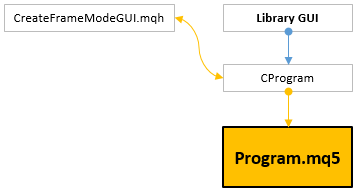### 开发用于处理帧数据的类

• 在第一种情况下，为了在图形对象上显示帧，需将指向这些对象的指针传递给类方法。
• 在第二种情况下，我们使用特殊方法接收帧数据以便填充其它类别的表格。

EasyAndFast 函数库采用来自标准库的 CGraphic 类将数据可视化。 我们将 它包含在 FrameGenerator.mqh 当中来访问其方法。

```//+------------------------------------------------------------------+
//|                                               FrameGenerator.mqh |
//|                                  版权所有 2018, MetaQuotes 软件公司 |
//|                                              http://www.mql5.com |
//+------------------------------------------------------------------+
#include <Graphics\Graphic.mqh>
//+------------------------------------------------------------------+
//| 用于接收优化结果的类                                                 |
//+------------------------------------------------------------------+
class CFrameGenerator
{
};
```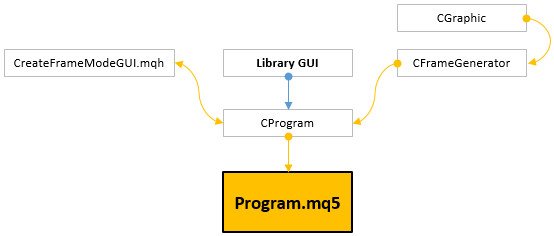• 第一个图形 (graph_balance) 显示优化结果余额的最后一连串指定数量。
• 第二个图形 (graph_result) 显示整体优化结果。
```class CFrameGenerator
{
private:
//--- 数据可视化的图形指针
CGraphic         *m_graph_balance;
CGraphic         *m_graph_results;
//---
public:
//--- 策略测试器事件处理程序
void              OnTesterEvent(const double on_tester_value);
void              OnTesterInitEvent(CGraphic *graph_balance,CGraphic *graph_result);
void              OnTesterDeinitEvent(void);
bool              OnTesterPassEvent(void);
};
//+------------------------------------------------------------------+
//| 应在 OnTesterInit() 处理器中调用                                    |
//+------------------------------------------------------------------+
void CFrameGenerator::OnTesterInitEvent(CGraphic *graph_balance,CGraphic *graph_results)
{
m_graph_balance =graph_balance;
m_graph_results =graph_results;
}
```

CFrameGenerator::OnTesterEvent() 方法中，我们得到测试结果余额和统计参数。 这些数据使用 CFrameGenerator::GetBalanceData() 和 CFrameGenerator::GetStatData() 方法传递给帧。 CFrameGenerator::GetBalanceData() 方法接收整个测试历史并汇总所有 in-/inout 交易。 得到的结果将逐步保存到 m_balance[] 数组中。 这个数组实际上还是 CFrameGenerator 类的成员。

```//--- 统计参数数量
#define STAT_TOTAL 7
//+------------------------------------------------------------------+
//| 用于处理优化结果的类                                                 |
//+------------------------------------------------------------------+
class CFrameGenerator
{
private:
//--- 结果余额
double            m_balance[];
//---
private:
//--- 接收余额数据
int               GetBalanceData(void);
//--- 接收统计数据
void              GetStatData(double &dst_array[],double on_tester_value);
};
//+------------------------------------------------------------------+
//| 获取余额数据                                                       |
//+------------------------------------------------------------------+
int CFrameGenerator::GetBalanceData(void)
{
int    data_count      =0;
double balance_current =0;
//--- 请求所有交易历史
::HistorySelect(0,LONG_MAX);
uint deals_total=::HistoryDealsTotal();
//--- 收集交易数据
for(uint i=0; i<deals_total; i++)
{
//--- 接收单号
ulong ticket=::HistoryDealGetTicket(i);
if(ticket<1)
continue;
//--- 如果是初始余额或 out/inout 交易
long entry=::HistoryDealGetInteger(ticket,DEAL_ENTRY);
if(i==0 || entry==DEAL_ENTRY_OUT || entry==DEAL_ENTRY_INOUT)
{
double swap      =::HistoryDealGetDouble(ticket,DEAL_SWAP);
double profit    =::HistoryDealGetDouble(ticket,DEAL_PROFIT);
double commision =::HistoryDealGetDouble(ticket,DEAL_COMMISSION);
//--- 计算余额
balance_current+=(profit+swap+commision);
//--- 保存到数组
data_count++;
::ArrayResize(m_balance,data_count,100000);
m_balance[data_count-1]=balance_current;
}
}
//--- 获取数据量
return(data_count);
}
//+------------------------------------------------------------------+
//| 接收统计数据                                                       |
//+------------------------------------------------------------------+
void CFrameGenerator::GetStatData(double &dst_array[],double on_tester_value)
{
::ArrayResize(dst_array,::ArraySize(m_balance)+STAT_TOTAL);
::ArrayCopy(dst_array,m_balance,STAT_TOTAL,0);
//--- 用测试结果填写第一个数组值 (STAT_TOTAL)
dst_array =::TesterStatistics(STAT_PROFIT);               // 净盈利
dst_array =::TesterStatistics(STAT_PROFIT_FACTOR);        // 盈利因子
dst_array =::TesterStatistics(STAT_RECOVERY_FACTOR);      // 恢复因子
dst_array =::TesterStatistics(STAT_DEALS);                // 成交数量
dst_array =::TesterStatistics(STAT_EQUITY_DDREL_PERCENT); // 最大资金回撤 %
dst_array =on_tester_value;                               // 自定义优化条件值
}
```

CFrameGenerator::GetBalanceData()CFrameGenerator::GetStatData() 方法在测试完成事件处理器中调用 — CFrameGenerator::OnTesterEvent()。 已接收数据。 将它们按帧发送到终端。

```//+------------------------------------------------------------------+
//| 准备余额值数组并将其在一个帧中发送                                      |
//| 函数应在 EA 的 OnTester() 处理器中调用                                |
//+------------------------------------------------------------------+
void CFrameGenerator::OnTesterEvent(const double on_tester_value)
{
//--- 获取余额数据
int data_count=GetBalanceData();
//--- 用于将数据发送到帧的数组
double stat_data[];
GetStatData(stat_data,on_tester_value);
//--- 用数据创建一个帧并将其发送到终端
::Print(__FUNCTION__," > 帧添加错误: ",::GetLastError());
else
::Print(__FUNCTION__," > 帧已添加");
}
```

```class CFrameGenerator
{
private:
//--- 处理帧的变量
string            m_name;
ulong             m_pass;
long              m_id;
double            m_value;
double            m_data[];
};
```

CFrameGenerator::SaveStatData() 方法从我们在帧中接收的数组中获取统计参数并将其保存到 单独的字符串数组 中。 那里的数据将包含指标名称和其值。 '=' 符号被用作分隔符。

```class CFrameGenerator
{
private:
//--- 含有统计参数的数组
string            m_stat_data[];
//---
private:
//--- 保存统计数据
void              SaveStatData(void);
};
//+------------------------------------------------------------------+
//| 将结果统计参数保存到数组中                                            |
//+------------------------------------------------------------------+
void CFrameGenerator::SaveStatData(void)
{
//--- 接收帧统计参数的数组
double stat[];
::ArrayCopy(stat,m_data,0,0,STAT_TOTAL);
::ArrayResize(m_stat_data,STAT_TOTAL);
//--- 填充测试结果数组
m_stat_data ="Net profit="+::StringFormat("%.2f",stat);
m_stat_data ="Profit Factor="+::StringFormat("%.2f",stat);
m_stat_data ="Factor Recovery="+::StringFormat("%.2f",stat);
m_stat_data ="Deals="+::StringFormat("%G",stat);
m_stat_data ="Equity DD="+::StringFormat("%.2f%%",stat);
m_stat_data ="OnTester()="+::StringFormat("%G",stat);
}
```

```class CFrameGenerator
{
public:
//--- 获取传递数组的统计参数
int               CopyStatData(string &dst_array[]) { return(::ArrayCopy(dst_array,m_stat_data)); }
};
```

```//--- 数组的待定尺寸
#define RESERVE_FRAMES 1000000
//+------------------------------------------------------------------+
//| 用于处理优化结果的类                                                 |
//+------------------------------------------------------------------+
class CFrameGenerator
{
private:
//--- 帧计数器
ulong             m_frames_counter;
//--- 有关正数和负数结果的数据
double            m_loss_x[];
double            m_loss_y[];
double            m_profit_x[];
double            m_profit_y[];
//---
private:
//--- 添加 (1) 负数和 (2) 正数结果至数组
};
//+------------------------------------------------------------------+
//| 添加负数结果至数组                                                  |
//+------------------------------------------------------------------+
{
int size=::ArraySize(m_loss_y);
::ArrayResize(m_loss_y,size+1,RESERVE_FRAMES);
::ArrayResize(m_loss_x,size+1,RESERVE_FRAMES);
m_loss_y[size] =loss;
m_loss_x[size] =(double)m_frames_counter;
}
//+------------------------------------------------------------------+
//| 添加正数结果至数组                                                   |
//+------------------------------------------------------------------+
{
int size=::ArraySize(m_profit_y);
::ArrayResize(m_profit_y,size+1,RESERVE_FRAMES);
::ArrayResize(m_profit_x,size+1,RESERVE_FRAMES);
m_profit_y[size] =profit;
m_profit_x[size] =(double)m_frames_counter;
}
```

```class CFrameGenerator
{
private:
//--- 更新结果图形
void              UpdateResultsGraph(void);
//--- 更新余额图形
void              UpdateBalanceGraph(void);
};
```

CFrameGenerator::UpdateResultsGraph() 方法中，将测试结果 (正/负利润) 添加到数组中。然后，这些数据显示在相应的图形上。 图形序列的名称 显示当前正数和负数结果的数量。

```//+------------------------------------------------------------------+
//| 更新结果图形                                                       |
//+------------------------------------------------------------------+
void CFrameGenerator::UpdateResultsGraph(void)
{
//--- 负数结果
if(m_data<0)
//--- 正数结果
else
//--- 在优化结果图形上更新序列
CCurve *curve=m_graph_results.CurveGetByIndex(0);
curve.Name("P: "+(string)ProfitsTotal());
curve.Update(m_profit_x,m_profit_y);
//---
curve=m_graph_results.CurveGetByIndex(1);
curve.Name("L: "+(string)LossesTotal());
curve.Update(m_loss_x,m_loss_y);
//--- 水平轴属性
CAxis *x_axis=m_graph_results.XAxis();
x_axis.Min(0);
x_axis.Max(m_frames_counter);
x_axis.DefaultStep((int)(m_frames_counter/8.0));
//--- 更新图形
m_graph_results.CalculateMaxMinValues();
m_graph_results.CurvePlotAll();
m_graph_results.Update();
}
```

CFrameGenerator::UpdateBalanceGraph() 方法的开始处，从帧中传递的数据数组中提取与余额相关的数据。 由于可以在图形上显示若干序列，我们应该令序列更新一致。 为达此目的，我们将使用一个单独的 序列计数器。 若要配置图形中同时显示的余额序列数量，我们需要 CFrameGenerator::SetCurvesTotal() 公共方法。 一旦序列计数器达到设定的极限，计数从头 开始。 帧计数器用作序列名称。 序列颜色也取决于结果: 绿色代表正面的结果，红色 — 代表负面的结果。

```class CFrameGenerator
{
private:
//--- 序列数量
uint              m_curves_total;
//--- 图上的当前序列索引
uint              m_last_serie_index;
//--- 定义最大序列
double            m_curve_max[];
//---
public:
//--- 设置要在图表上显示的序列数量
void              SetCurvesTotal(const uint total);
};
//+------------------------------------------------------------------+
//| 设置要在图表上显示的序列数量                                          |
//+------------------------------------------------------------------+
void CFrameGenerator::SetCurvesTotal(const uint total)
{
m_curves_total=total;
::ArrayResize(m_curve_max,total);
::ArrayInitialize(m_curve_max,0);
}
//+------------------------------------------------------------------+
//| 更新余额图                                                         |
//+------------------------------------------------------------------+
void CFrameGenerator::UpdateBalanceGraph(void)
{
//--- 接收当前帧的余额值的数组
double serie[];
::ArrayCopy(serie,m_data,0,STAT_TOTAL,::ArraySize(m_data)-STAT_TOTAL);
//--- 发送数组以便在余额图上显示
CCurve *curve=m_graph_balance.CurveGetByIndex(m_last_serie_index);
curve.Name((string)m_frames_counter);
curve.Color((m_data>=0)? ::ColorToARGB(clrLimeGreen) : ::ColorToARGB(clrRed));
curve.Update(serie);
//--- 获取序列大小
int serie_size=::ArraySize(serie);
m_curve_max[m_last_serie_index]=serie_size;
//--- 定义具有最大元素数两的序列
double x_max=0;
for(uint i=0; i<m_curves_total; i++)
x_max=::fmax(x_max,m_curve_max[i]);
//--- 水平轴属性
CAxis *x_axis=m_graph_balance.XAxis();
x_axis.Min(0);
x_axis.Max(x_max);
x_axis.DefaultStep((int)(x_max/8.0));
//--- 更新结果图
m_graph_balance.CalculateMaxMinValues();
m_graph_balance.CurvePlotAll();
m_graph_balance.Update();
//--- 增加序列计数器
m_last_serie_index++;
//--- 如果达到极限，请将序列计数器设置为零
if(m_last_serie_index>=m_curves_total)
m_last_serie_index=0;
}
```

```class CFrameGenerator
{
private:
//--- EA 参数
string            m_param_data[];
uint              m_par_count;
};
//+------------------------------------------------------------------+
//| 在优化过程中接收数据帧并显示图形                                       |
//+------------------------------------------------------------------+
bool CFrameGenerator::OnTesterPassEvent(void)
{
//--- 获取新帧后，尝试从中提取数据
if(::FrameNext(m_pass,m_name,m_id,m_value,m_data))
{
//--- 获取所形成帧的 EA 输入参数
::FrameInputs(m_pass,m_param_data,m_par_count);
//--- 将结果统计参数保存到数组中
SaveStatData();
//--- 更新结果和余额图
UpdateResultsGraph();
UpdateBalanceGraph();
//--- 增加处理帧的计数器
m_frames_counter++;
return(true);
}
//---
return(false);
}
```

```class CFrameGenerator
{
private:
//--- 释放数组
void              ArraysFree(void);
//--- 优化后所有帧的最终数据重新计算
void              FinalRecalculateFrames(void);
};
//+------------------------------------------------------------------+
//| 释放数组                                                           |
//+------------------------------------------------------------------+
void CFrameGenerator::ArraysFree(void)
{
::ArrayFree(m_loss_y);
::ArrayFree(m_loss_x);
::ArrayFree(m_profit_y);
::ArrayFree(m_profit_x);
}
//+------------------------------------------------------------------+
//| 优化后所有帧的最终数据重新计算                                         |
//+------------------------------------------------------------------+
void CFrameGenerator::FinalRecalculateFrames(void)
{
//--- 将帧指针设置到开始
::FrameFirst();
//--- 重置计数器和数组
ArraysFree();
m_frames_counter=0;
//--- 启动循环遍历帧
while(::FrameNext(m_pass,m_name,m_id,m_value,m_data))
{
//--- 负数结果
if(m_data<0)
//--- 正数结果
else
//--- 增加处理帧的计数器
m_frames_counter++;
}
//--- 在图形上更新序列
CCurve *curve=m_graph_results.CurveGetByIndex(0);
curve.Name("P: "+(string)ProfitsTotal());
curve.Update(m_profit_x,m_profit_y);
//---
curve=m_graph_results.CurveGetByIndex(1);
curve.Name("L: "+(string)LossesTotal());
curve.Update(m_loss_x,m_loss_y);
//--- 水平轴属性
CAxis *x_axis=m_graph_results.XAxis();
x_axis.Min(0);
x_axis.Max(m_frames_counter);
x_axis.DefaultStep((int)(m_frames_counter/8.0));
//--- 更新结果图
m_graph_results.CalculateMaxMinValues();
m_graph_results.CurvePlotAll();
m_graph_results.Update();
}
```

```//+------------------------------------------------------------------+
//| 应在 OnTesterDeinit() 处理器中调用                                  |
//+------------------------------------------------------------------+
void CFrameGenerator::OnTesterDeinitEvent(void)
{
//--- 优化后最终重新计算所有帧的数据
FinalRecalculateFrames();
//--- 记住总帧数并将计数器设置为零
m_frames_total     =m_frames_counter;
m_frames_counter   =0;
m_last_serie_index =0;
}
```

### 在应用程序类中处理数据优化

```class CProgram : public CWndEvents
{
private:
//--- 界面可用性
void              IsAvailableGUI(const bool state);
void              IsLockedGUI(const bool state);
}
//+------------------------------------------------------------------+
//| 优化开始事件                                                       |
//+------------------------------------------------------------------+
void CProgram::OnTesterInitEvent(void)
{
//--- 创建图形界面
if(!CreateFrameModeGUI())
{
::Print(__FUNCTION__," > 不能创建图形界面！");
return;
}
//--- 令界面无法访问
IsLockedGUI(false);
//--- 初始化帧生成器
m_frame_gen.OnTesterInitEvent(m_graph1.GetGraphicPointer(),m_graph2.GetGraphicPointer());
}
//+------------------------------------------------------------------+
//| 界面可用性                                                         |
//+------------------------------------------------------------------+
void CProgram::IsAvailableGUI(const bool state)
{
m_window1.IsAvailable(state);
m_sleep_ms.IsAvailable(state);
m_curves_total.IsAvailable(state);
}
//+------------------------------------------------------------------+
//| 阻塞界面                                                          |
//+------------------------------------------------------------------+
void CProgram::IsLockedGUI(const bool state)
{
m_window1.IsAvailable(state);
m_sleep_ms.IsLocked(!state);
m_curves_total.IsLocked(!state);
}
```

```class CProgram : public CWndEvents
{
private:
//--- 更新统计表
//--- 更新参数表
void              UpdateParamTable(void);
}
//+------------------------------------------------------------------+
//| 更新统计表                                                         |
//+------------------------------------------------------------------+
{
//--- 获取统计表的数据数组
string stat_data[];
int total=m_frame_gen.CopyStatData(stat_data);
for(int i=0; i<total; i++)
{
//--- 分成两行并输入到表格
string array[];
if(::StringSplit(stat_data[i],'=',array)==2)
{
if(m_frame_gen.CurrentFrame()>1)
m_table_stat.SetValue(1,i,array,0,true);
else
{
m_table_stat.SetValue(0,i,array,0,true);
m_table_stat.SetValue(1,i,array,0,true);
}
}
}
//--- 更新表格
m_table_stat.Update();
}
```

```//+------------------------------------------------------------------+
//| 优化通关处理事件                                                    |
//+------------------------------------------------------------------+
void CProgram::OnTesterPassEvent(void)
{
//--- 处理获得的测试结果并显示图形
if(m_frame_gen.OnTesterPassEvent())
{
UpdateParamTable();
}
}
```

```class CProgram : public CWndEvents
{
private:
//--- 计算正数和负数结果的比率
void              CalculateProfitsAndLosses(void);
}
//+------------------------------------------------------------------+
//| 计算正数和负数结果的比率                                              |
//+------------------------------------------------------------------+
void CProgram::CalculateProfitsAndLosses(void)
{
//--- 如果没有帧，退出
if(m_frame_gen.FramesTotal()<1)
return;
//--- 负数和正数结果的数量
int losses  =m_frame_gen.LossesTotal();
int profits =m_frame_gen.ProfitsTotal();
//--- 百分比
string pl =::DoubleToString(((double)losses/(double)m_frame_gen.FramesTotal())*100,2);
string pp =::DoubleToString(((double)profits/(double)m_frame_gen.FramesTotal())*100,2);;
//--- 显示在状态栏中
m_status_bar.SetValue(1,"Profits: "+(string)profits+" ("+pp+"%)"+" / Losses: "+(string)losses+" ("+pl+"%)");
m_status_bar.GetItemPointer(1).Update(true);
}
```

```//+------------------------------------------------------------------+
//| 优化完成事件                                                       |
//+------------------------------------------------------------------+
void CProgram::OnTesterDeinitEvent(void)
{
//--- 优化完成
m_frame_gen.OnTesterDeinitEvent();
//--- 令界面可访问
IsLockedGUI(true);
//--- 计算正数和负数结果的比率
CalculateProfitsAndLosses();
//--- 初始化图形界面核心
CWndEvents::InitializeCore();
}
```

• 输入字段中的变化用于设置图形上显示的余额序列的数量;
• 启动查看优化结果。

CProgram::UpdateBalanceGraph() 方法用于更改序列数量后更新图形。 此处我们设置在帧生成器中工作的序列数量，然后在图形上保留这个数字。

```class CProgram : public CWndEvents
{
private:
//--- 更新结果图
void              UpdateBalanceGraph(void);
};
//+------------------------------------------------------------------+
//| 更新图形                                                           |
//+------------------------------------------------------------------+
void CProgram::UpdateBalanceGraph(void)
{
//--- 设置工作序列的数量
int curves_total=(int)m_curves_total.GetValue();
m_frame_gen.SetCurvesTotal(curves_total);
//--- 删除序列
CGraphic *graph=m_graph1.GetGraphicPointer();
int total=graph.CurvesTotal();
for(int i=total-1; i>=0; i--)
graph.CurveRemoveByIndex(i);
//--- 添加序列
double data[];
for(int i=0; i<curves_total; i++)
//--- 更新结果图
graph.CurvePlotAll();
graph.Update();
}
```

```//+------------------------------------------------------------------+
//| 事件处理器                                                         |
//+------------------------------------------------------------------+
void CProgram::OnEvent(const int id,const long &lparam,const double &dparam,const string &sparam)
{
//--- 按钮触发事件
if(id==CHARTEVENT_CUSTOM+ON_CLICK_BUTTON)
{
//--- 更改图表上的序列数量
if(lparam==m_curves_total.Id())
{
UpdateBalanceGraph();
return;
}
return;
}
//--- 在输入字段中输入数值的事件
if(id==CHARTEVENT_CUSTOM+ON_END_EDIT)
{
//--- 更改图表上的序列数量
if(lparam==m_curves_total.Id())
{
UpdateBalanceGraph();
return;
}
return;
}
}
```

```class CFrameGenerator
{
public:
//--- 循环遍历帧
bool              ReplayFrames(void);
};
//+------------------------------------------------------------------+
//| 优化完成后重演帧                                                    |
//+------------------------------------------------------------------+
bool CFrameGenerator::ReplayFrames(void)
{
//--- 将帧指针设置为开头
if(m_frames_counter<1)
{
ArraysFree();
::FrameFirst();
}
//--- 启动循环遍历帧
if(::FrameNext(m_pass,m_name,m_id,m_value,m_data))
{
//--- 获取 EA 输入，哪个帧已经形成
::FrameInputs(m_pass,m_param_data,m_par_count);
//--- 将统计结果参数保存到数组中
SaveStatData();
//--- 更新结果和余额图
UpdateResultsGraph();
UpdateBalanceGraph();
//--- 增加处理帧的计数器
m_frames_counter++;
return(true);
}
//--- 循环完成
m_frames_counter   =0;
m_last_serie_index =0;
return(false);
}
```

CFrameGenerator::ReplayFrames() 方法在 CProgram 类中由 ViewOptimizationResults() 方法调用。 在启动帧之前，图形界面变得不可用。 可在 Sleep 输入字段中指定暂停来调整滚动速度。 同时，状态栏会显示 进度条，示意进程结束前的时间。

```class CFrameGenerator
{
private:
//--- 查看优化结果
void              ViewOptimizationResults(void);
};
//+------------------------------------------------------------------+
//| 查看优化结果                                                        |
//+------------------------------------------------------------------+
void CProgram::ViewOptimizationResults(void)
{
//--- 令界面不可访问
IsAvailableGUI(false);
//--- 暂停
int pause=(int)m_sleep_ms.GetValue();
//--- 播放帧
while(m_frame_gen.ReplayFrames() && !::IsStopped())
{
//--- 更新表格
UpdateParamTable();
//--- 更新进度条
m_progress_bar.Show();
m_progress_bar.LabelText("Replay frames: "+string(m_frame_gen.CurrentFrame())+"/"+string(m_frame_gen.FramesTotal()));
m_progress_bar.Update((int)m_frame_gen.CurrentFrame(),(int)m_frame_gen.FramesTotal());
//--- 暂停
::Sleep(pause);
}
//--- 计算正数和负数结果的比率
CalculateProfitsAndLosses();
//--- 隐藏进度条
m_progress_bar.Hide();
//--- 令界面可用
IsAvailableGUI(true);
}
```

CProgram::ViewOptimizationResults() 方法通过在应用程序图形界面上按重演帧 按钮来调用。 ON_CLICK_BUTTON 事件生成。

```//+------------------------------------------------------------------+
//| 事件处理器                                                         |
//+------------------------------------------------------------------+
void CProgram::OnEvent(const int id,const long &lparam,const double &dparam,const string &sparam)
{
//--- 按下按钮的事件
if(id==CHARTEVENT_CUSTOM+ON_CLICK_BUTTON)
{
//--- 查看优化结果
{
ViewOptimizationResults();
return;
}
//---
...
return;
}
}
```

### 显示获得的结果

FormatString.mqh 在这里作为一个附加函数用于行格式化。 它们不是任何类的一员，所以我们用黑色标记箭头。 由此产生的应用程序结构如下所示: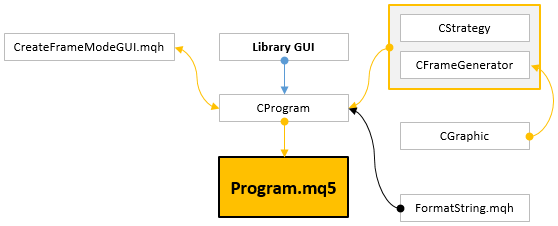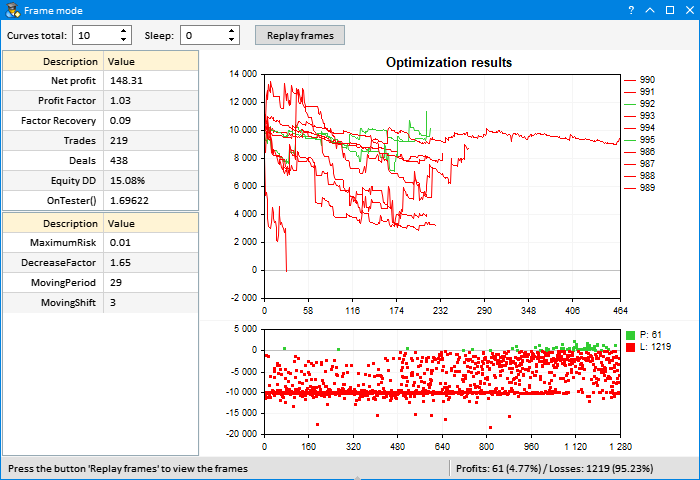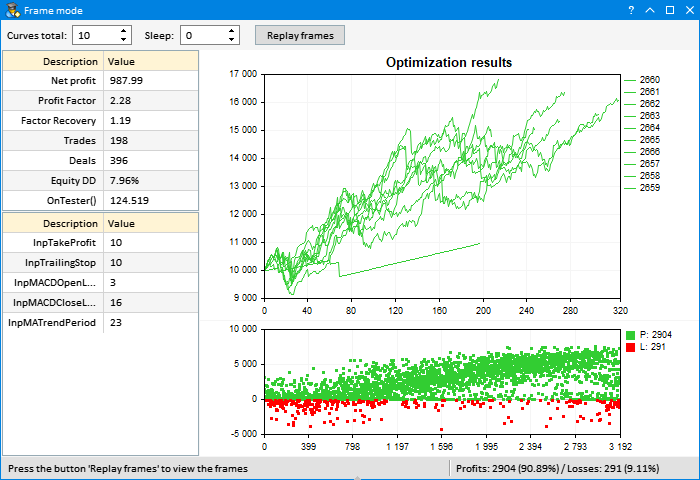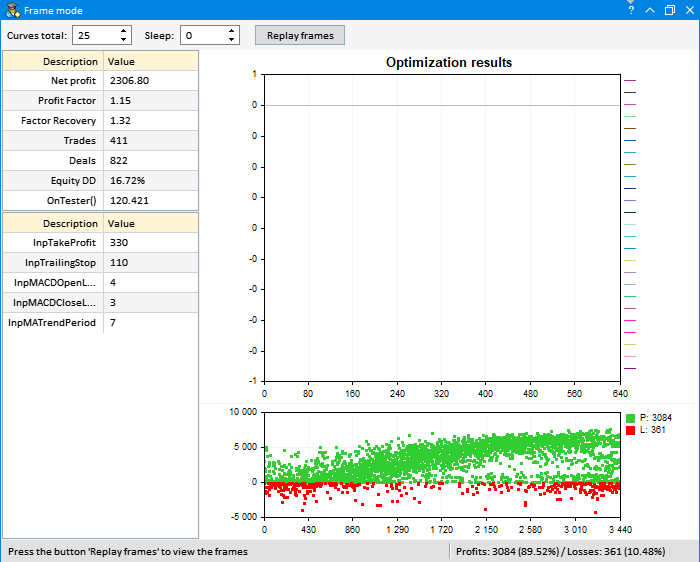### 结束语

MQL5.zip (33.34 KB)

#### 该作者的其他文章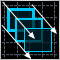如何创建任意复杂度的图形面板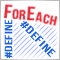交易者生活窍门: 利用 defines (#define) 融合 ForEach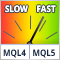自置缓存的指标速度比较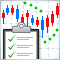如何创建订购指标的需求规范